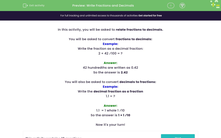# Write Fractions and Decimals

In this worksheet, students will be asked to relate fractions to their decimal equivalents.Key stage:  KS 2

Curriculum topic:   Number: Fractions, Decimals and Percentages

Curriculum subtopic:   Relate Fractions to Decimals

Difficulty level:#### Worksheet Overview

In this activity, you will be asked to relate fractions to decimals.

You will be asked to convert fractions to decimals:

Example:

Write the fraction as a decimal:

2 + 42 ⁄100 = ?

42 hundredths are written as 0.42

You will also be asked to convert decimals to fractions:

Example:

Write the decimal as a fraction

1.1 = ?

1.1  = 1 whole 1 ⁄10

So the answer is 1 + 1 ⁄10